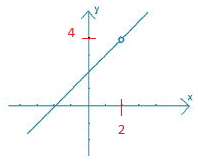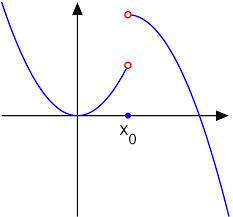Types of Discontinuities IIT JEE

Types of Discontinuity - Properties of Discontinuous Functions

Discontinuity of a function can be defined as the point at which the continuity of a particular function cannot be defined in its current domain. While continuity of a particular function is very important in the study of mathematics, similarly discontinuity of a function is also very important in mathematics, its applications and in its functions. The discontinuity of a function can be defined through the set of points, discrete set along with the dense set and even with the complete domain of the function. The removable type of discontinuity is the only type of discontinuity which is fixed and can be redefined, while all other types of discontinuities are designated by the fact that the limit does not exist and cannot be redefined further.

Continuity and discontinuity of a function can be explained with the help of the following example:

A function f(x) can be said as continuous at a particular point of x =a, if

lim xa- f(x) = lim xa+ f(x) = f(a)

In the above equation, the function f(x) can be said as continuous at the particular set of point only when the limiting value of the given function is equal to the value of f(a), and the function is said to be continuous at the point of x =a.

But the function is said to be discontinuous at the point x =a, while having the following conditions:

• If the limiting value of xa- f(x) and the limiting value of xa+ f(x) exists but are not having the same value, then the function is said to be discontinuous.
• If the limiting value of xa- f(x) and the limiting value of xa+ f(x) exist and have the same value but are not equal to the value of f(a) then the function is said to be discontinuous.
• At least one or more than one of the limit does not exist then also the function is said to be discontinuous.
• If the function f(a) is not defined, then in this condition the function is said to be discontinuous.
• Every rational number is continuous except for having its denominator 0, as it becomes discontinuous with the denominator being 0.

Some properties of a function being discontinuous

There are many properties which show a function to be discontinuous but two most important properties include as follows:

• The limit of the function at a discontinuous point and even in the most discontinuous function is undefined. However, this is not true in some cases as the limit can be redefined but still it is said to be discontinuous.
• For all the cases, the function can break-off at a particular point or at multiple points as the discontinuous functions have points at which they break-off and picked up from another location.

Types of discontinuities

Various types of discontinuities can occur in the function which can be easily distinguished between one another at a given function f. This can be shown as follows:

1. Removable Discontinuity
• Missing Point Discontinuity
• Isolated Point Discontinuity
2. Non- removable Discontinuity
• Finite Type Discontinuity
• Infinite Type Discontinuity
• Oscillatory Discontinuity

1. Removable Discontinuity:

Removable discontinuity is a type of discontinuity in which the limit of a function f(x) certainly exists but having the problem of either having the different value of both the function f(x) and f(a) or it does not have a defined value of the function f(a). But in removable discontinuity, there is a possibility of having the value of a function and the value of limit equal to each other at a given particular point i.e. lim xa f(x) =f(a).

The removable discontinuity can be easily explained with the help of the following example;

When the function f(x) = [x] + [-x] is redefined in such a way that it becomes continuous for ∈ (0, 2) then this type of discontinuity is referred to as removable discontinuity.

Solution-

Here lim x→1 f(x) = -1 but with f(a) = 0

Hence, f(x) consists of a removable discontinuity at point x = 1.

To remove this discontinuity, we define f(x) as follows:

f(x) = [x] + [-x], x ∈ (0, 1) U (1, 2)

f(x) = -1

Where, x = 1.

Now, the function f(x) is continuous for x ∈ (0, 2).

This removable type of discontinuity can be further divided into types which are described as missing point type and isolated point type of discontinuity.

• Missing Point Discontinuity;

The missing point discontinuity is that type of discontinuity in which the limit of the function exists at a particular point ‘a’ but the function f(a) is not defined at that particular point ‘a’. This type of missing point discontinuity can be explained with the help of the following example:

Example-

To find the value of missing point discontinuity in the function f(x) = (x2 - 4) / (x – 2), where x ≠ 2, we can look through the following graph:Through the graph shown above, it is easily visible that f(2) is not defined and hence the value of missing point of discontinuity of f(x) is at the point x = 2.

• Isolated Point Discontinuity;

The isolated point discontinuity is that type of discontinuity, in which the limit of the function f(x) exists at the particular point ‘a’ along with the function f(a) which is also defined at that particular point ‘a’, but the values of both the terms are not equal i.e.
lim xa f(x) ≠ f(a).

Example-

Through the following example, we can find out the value of isolated point type of discontinuity in any function;
f(x) = sgn (cos 2x – 2sin x + 3),
f(x) = sgn (2 (2 + sin x)(1 – sin x)) = 0, if x = 2nπ + π/2
And, f(x) = sgn (2 (2 + sin x)(1 – sin x)) = +1, if x ≠ 2nπ + π/2
Through the above given example we can say that the isolated point is at x=0, in which discontinuity occurs as x = 2nπ + π/2.

2. Non-removable Discontinuity:

Non-removable discontinuity is the type of discontinuity in which the limit of the function does not exist at a given particular point i.e. lim xa f(x) does not exist. We can simply say that the value of f(a) at the function with x = a (which is the point of discontinuity) may or may not exist but the limit xa f(x) does not exist. This non-removable type of discontinuities can be further divided into three types which are finite type, infinite type and oscillatory type of discontinuities.

• Finite Type Discontinuity;

In this type of discontinuity, despite of finitely existing f(a), the function can be either equal to lim xa- f(x) or lim xa+ f(x), as both the sides of a function including {lim xa- f(x) & lim xa+ f(x)} are not equal. This type of function having the non-negative difference between the two limits can be said as a jump of discontinuity. This type of discontinuity can be easily explained with the help of the following examples:

Example-

In the function f(x) = [x], where [x] is the greatest integer < x.
Take x = 1, where 1 is some integer.
In which, RHL = lim x→1+ f(x) = 1 and,
LHL = lim x→a- f(x) = 1-1
This shows that both RHL and LHL exist and have some finite value but are not equal to each other.In the figure shown above, the two points show the jump of discontinuity which is having finite value in a finite interval. It can also be stated as a function having a finite number of jumps in the given finite amount of interval can be called a piecewise continuous function.

• Infinite Type Discontinuity;

Infinite type of discontinuity is that type of discontinuity in which either RHL or LHL, one of the limits tends to move to infinity. In this type of discontinuity, both lim xa f(x) and f(a) do not exist, having one of the limits till infinity. The infinite discontinuity can be explained with the help of the following example:

Example-

For, f(x) = 1/ (x - 4), where f(4) is undefined.
1/ (x – 4) -∞, for x 4-
And, 1/ (x 4) ∞, for x 4+
From the above example, it is clear that x = 4 is the point of discontinuity occurring at ∞, since both the sides of the line x = 4 tends to ∞.

• Oscillatory Type Discontinuity;

Oscillatory type of discontinuity is the type of discontinuity in which limits oscillate between two values which are finite. It can be divided into two types as, if f(a) is undefined at a function f having an oscillatory finite function f(x) x a then, x = a can be said as the point of finite oscillatory discontinuity. While, in the case of an oscillatory infinite function f(x) x a, then x = a can be called as the point of infinite oscillatory discontinuity.